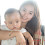## Introduction

In our previous post, we talked about adding and subtracting decimals. Well, in this post we will learn how to multiply and divide decimals. So, let's get started!

## Multiplying Decimals

Let's say that we have the two decimals 5.8 x 6.2. The first step is to rewrite this in proper multiplication format:

5.8
x 6.2

The next step is just simple multiplication. ( Forget the decimals point for now...)

58
x 62
3596

Now, count the number of digits after the decimals points for each of the numbers:

5.8= 1 digit
6.2= 1 digit

So, there is a total of 2 digits.
Now, move the decimals point in 3596 two places from right to left:

3596.0
35.96

So that means the product of 5.8 and 6.2 equals 35.96!

## Dividing Decimals

In order to divide decimals you need to change the divisor into a whole number by moving the decimal point.

Let's say we have the problem 2.46/1.2.
The divisor, 1.2, will move 1 place  from left to right and become a whole number of 12.
Remember that you have to do the same thing to the dividend as you did to the divisor. So, you will move the decimal point in 2.46 one place from left to right:
2.46 becomes 24.6.

24.6/12

Now just simply divide!

24.6/12=2.05

So that means the quotient for 2.46/1.2=2.05!

Sheet 1 How to Multiply and Divide Decimals

#### 1 comment:

1.Adding and Subtracting numbers is time consuming and can exhaust too much energy on thinking what is the right answer. If practicing how to add and subtract numbers, it is better to do it manually but when you are in an actual situation that you are doing arithmetic operation, it is better to use calculator. Using calculator is not bad and it is a good strategy to save your time and physical strength plus you can be sure that you got the correct answer.